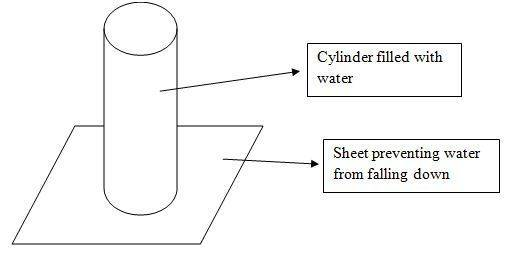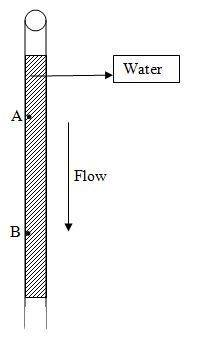# Bernoulli and Continuity Equations

bgq
Hi,
Consider a vertical relatively long cylinder of constant radius open at both ends. We fill this cylinder with water and prevent water from falling down by a certain sheet as seen in the figure.Now suppose we remove the sheet suddenly. Let v1 be the speed of the upper surface of water, and v2 be the speed of the water at the bottom of the cylinder.

According to the equation of continuity A1v1 = A2v2, since the cylinder has constant radius then A1=A2 and so v1 = v2.
According to Bernoulli's equation: P1 + 0.5pv12 + pgh1 = P2 + 0.5pv22 + pgh2
Setting P1 = P2 = P0 (of atmosphere), h1 - h2 = H, we get v22 = v12 + pgH which states clearly that v2 > v1

Question: Which one is correct and why?

Gold Member
and v2 be the speed of the water at the bottom of the cylinder.

Due to molecular cohesion of water, the bottom of the water cylinder will not fall everywhere with the same velocity, but a ray will form which will get thinner as the water falls down, similar to water ray you can see falling down from the kitchen water tap. The pressure in the water varies with position, and only approaches the atmospheric pressure after it has travelled some distance from the orifice (bottom of the cylinder). Search for vena contracta, coefficient of contraction, efflux coefficient. In Feynman's lectures on physics, p. II, chapter on hydrodynamics 40-3, he gives great explanation why this happens.

Mentor
Hi,
Consider a vertical relatively long cylinder of constant radius open at both ends. We fill this cylinder with water and prevent water from falling down by a certain sheet as seen in the figure.

View attachment 62754

Now suppose we remove the sheet suddenly. Let v1 be the speed of the upper surface of water, and v2 be the speed of the water at the bottom of the cylinder.

According to the equation of continuity A1v1 = A2v2, since the cylinder has constant radius then A1=A2 and so v1 = v2.
According to Bernoulli's equation: P1 + 0.5pv12 + pgh1 = P2 + 0.5pv22 + pgh2
Setting P1 = P2 = P0 (of atmosphere), h1 - h2 = H, we get v22 = v12 + pgH which states clearly that v2 > v1

Question: Which one is correct and why?

The form of the Bernoulli equation you used is for a steady flow (time independent) system, but the the system you describe is not a steady flow process because things within the tube are changing with time (namely, the amount of liquid in the tube). You need to include the rate of change of mechanical energy of the liquid in the tube.

bgq
The form of the Bernoulli equation you used is for a steady flow (time independent) system, but the the system you describe is not a steady flow process because things within the tube are changing with time (namely, the amount of liquid in the tube). You need to include the rate of change of mechanical energy of the liquid in the tube.

But Suppose we consider two points in the tube A and B. We choose the system to be formed of the water between A and B. We study the motion of the water in the time interval when the upper surface of water is still above A as seen in the following figure.During this time interval, the flow is steady, so we can apply Bernoulli's equation to find that VB > VA, but the continuity equation will say that VA = VB

Mentor
The flow is not steady. The fluid contained between A and B is accelerating, even though, at any given time VA = VB. Both VA and VB are increasing with respect to time. If you take into account the rate of increase of kinetic energy of the fluid between A and B in the balance equation, it will resolve the issue.

Mentor
Let's do a force balance on the plug of fluid between locations A and B and any given time. The forces acting on this fluid plug are the pressure forces on its ends, and the weight of the plug. The sum of these forces must equal the mass of the plug times its acceleration.

$$A(z_A-z_B)ρ\frac{dV}{dt}=(P_A-P_B)A+A(z_A-z_B)ρg$$

where A is the cross sectional area of the cylinder, ρ is the fluid density, z is distance measured upward, and V is the downward fluid velocity throughout the plug (VA=VB=V). If we divide this equation by A, and rearrange, we obtain:

$$P_A+ρgz_A=P_B+ρgz_B+ρ(z_A-z_B)\frac{dV}{dt}$$

This is the form of the equation applicable to your unsteady state situation. Note that there are no terms for kinetic energy at the two ends of the plug, since the velocities are the same at the two ends.

Here's something even more interesting. If you apply this same analysis to the entire column of fluid within the cylinder at any time, since the pressures at the top and bottom of the overall plug are atmospheric, you find simply that, for your situation:
$$\frac{dV}{dt}=g$$

If you substitute this into the previous relationship (for cross sections A and B), you find that

PA=PB

Since the locations A and B are totally arbitrary, this shows that the pressure P at all locations along the vertical column of fluid is constant, and equal to atmospheric pressure. How can this be, since it is incompatible with the hydrostatic equation? Well, it tells you that the situation in your problem isn't anywhere close to hydrostatic equilibrium. What these equations are telling you is that the column of fluid within the cylinder is actually in free fall, so you wouldn't expect the hydrostatic equation to come close to applying.

•1 person
bgq
Let's do a force balance on the plug of fluid between locations A and B and any given time. The forces acting on this fluid plug are the pressure forces on its ends, and the weight of the plug. The sum of these forces must equal the mass of the plug times its acceleration.

$$A(z_A-z_B)ρ\frac{dV}{dt}=(P_A-P_B)A+A(z_A-z_B)ρg$$

where A is the cross sectional area of the cylinder, ρ is the fluid density, z is distance measured upward, and V is the downward fluid velocity throughout the plug (VA=VB=V). If we divide this equation by A, and rearrange, we obtain:

$$P_A+ρgz_A=P_B+ρgz_B+ρ(z_A-z_B)\frac{dV}{dt}$$

This is the form of the equation applicable to your unsteady state situation. Note that there are no terms for kinetic energy at the two ends of the plug, since the velocities are the same at the two ends.

Here's something even more interesting. If you apply this same analysis to the entire column of fluid within the cylinder at any time, since the pressures at the top and bottom of the overall plug are atmospheric, you find simply that, for your situation:
$$\frac{dV}{dt}=g$$

If you substitute this into the previous relationship (for cross sections A and B), you find that

PA=PB

Since the locations A and B are totally arbitrary, this shows that the pressure P at all locations along the vertical column of fluid is constant, and equal to atmospheric pressure. How can this be, since it is incompatible with the hydrostatic equation? Well, it tells you that the situation in your problem isn't anywhere close to hydrostatic equilibrium. What these equations are telling you is that the column of fluid within the cylinder is actually in free fall, so you wouldn't expect the hydrostatic equation to come close to applying.

This is extremely helpful, thank you very much